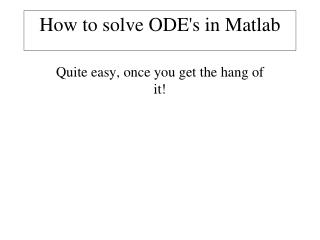DownloadDownload PresentationHow to solve ODE's in Matlab

# How to solve ODE's in Matlab

Download Presentation## How to solve ODE's in Matlab

- - - - - - - - - - - - - - - - - - - - - - - - - - - E N D - - - - - - - - - - - - - - - - - - - - - - - - - - -
##### Presentation Transcript

1. How to solve ODE's in Matlab Quite easy, once you get the hang of it!

2. Matlab has a series of solvers. • Most often, it is OK to us ODE23 or ODE45 • ode23 better for less smooth solutions • ode45 better for smoother solutions • "doc ode23" gives you information on all of them, but requires more math than I have presented to fully understand

3. ode45 and its friends are called as follows: • [T,Y] = ode45(@odefun,tspan,y0) • odefun(t,y) is a function! • Inputs: • t is the time, a scaler (explain scaler) • y is a column vector of the variables you are solving for • Output: • dy_dt, a column vector of the time derivative of y

4. Prey are born Prey are eaten, and turned into Carnivores Carnivores die of old age • Example, from our old prey/carnivore system: • y=[P;C] (why the ";"?) • dy_dt is

5. So what does the code for the derivative function look like? function dPopdt=dPop_dt(t,Pop); %calculate the Lotka-Volterra carnivore prey equations % % d(Prey)/dt = Prey*(alpha-beta*Carnivore) % d(Carnivore)/dt = -Carnivore*(gamma-delta*Prey) % % where % % The state variable is Pop(1) is prey, Pop(2) is carnivore gamma=0.05; delta=0.001; alpha=0.04; beta=0.00075; dPopdt=[1;1]; %Explain this line! dPopdt(1)=Pop(1)*(alpha-beta*Pop(2)); dPopdt(2)=-Pop(2)*(gamma-delta*Pop(1));

6. And we would call this with: • [T,Pop] = ode45(@dPop_dt,tspan,y0) • tspan=[tstart,tmin] • y0 is a column vector of the initial condition • ode45 returns • T, all the time it computed a solution for • Y, a matrix that has the same number of columns as y0 has rows and the same number of rows as T, which is the solution • each row corresponds to a time in T • each column corresponds to a variable • Yes, this is stupid. (why do I say this?)

7. Example: P0=[10;10]; %intial condition %use ode45 to solve [tvec,Pvec]=ode45(@dPop_dt,[0,500],P0); plot(tvec,Pvec(:,2),'r*-',tvec,Pvec(:,1),'g-','linewidth',2) xlabel('time, days') ylabel('critter numbers') title('Red are Predators, Green are Prey') grid on

8. In the lab we will study a radioactive decay series, were one element decays into another which decays into another: • What are the equations for this, given • Element E1, a fraction  of which decays each year to E2, • Element E2, a fraction  of which decays each year to E3, • and etc. • In units of moles (why?)

9. At “equilibrium”, what would the ratio of E1 to E2 be, roughly? • -- Why?

10. How to draw a legend on your plots • han=plot(t,x1,’r-’,t,x2,’b-’) • legend(han,’the red line’,’the blue line’)